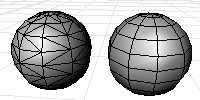Note: Diagonals that are unwelded (have unique vertexes at the corners) will not be considered as candidates for merging triangles into quadrangles.

Steps

Select mesh objects.

Options

Planarity

The angle between the face normals of the triangles.

Rectangularity

Specify a number greater than or equal to 1. If the adjacent triangles pass the planarity test then they will be merged into a quadrangle if the largest ratio of the lengths of the diagonal is less than or equal to the number you typed, then the triangles are converted to quadrangle.